# Post-processing and Statistical Checks for Random Numbers – C PROGRAM

In the last post I wrote about random numbers and how to generate them.

Now even though, the random number sequence generated from the algorithms I mentioned in the last post may seem truly random to our mind, we can’t be a 100% sure without performing some kind of a statistical check.

Now I already mentioned two ways to test our algorithm in the last post. And I am going to just write about the same checks here, so there is nothing new here if you read the last post. However, if you ended up here from google search then this might be useful for you.

Well, the first check would be to plot a distribution of random numbers. Let’s say your algorithm produces random numbers between 0 and 1. Then, ideally the number of random numbers generated in the windows$[0,0.1]$,$[0.1,0.2]$, etc. should be equal. Because there is no a priori reason for our algorithm to be preferring a particular number or range of numbers. Therefore, all numbers or ranges should be equally probable. Think of it this way. An unbiased coin, if tossed a lot of times, would give you almost the same number of Heads and Tails, thereby not preferring any particular outcome.

However, we will soon see that this test is not sufficient.

This brings me to another test, that is the correlation test.
For this, you could plot$r_{i+1}$ vs.$r_i$ and see if the graph shows any correlation.
Moreover, you could even repeat the process to see if there is any correlation between$r_{i+2}$ and$r_i$ ,$r_{i+3}$$r_i$, and so on.

The following programs will illustrate the process.
I will use a popular algorithm (formula) to generate random numbers, that is:$r_{i+1}=(a\times r_i)\mathrm{mod } m$
called the Linear Congruential Generator
This algorithm generates a maximum of$(m-1)$ random numbers with the maximum value of$m-1$ (Try to see why is it so).
Here,$r_i$ is the seed.
The values of$a$ and$m$ are carefully chosen values.

In this program I will scale down the random numbers to lie between$[0,1]$ by dividing them by$(m-1)$ as that is the largest random number that can be generated. Then I will find out the frequency distribution within windows of width 0.1, and store these in a .txt file. Then I’ll also do a correlation test, where I will store$r_{i+1}$ and$r_i$ in a .txt file and then plot them to see any correlation.

### CODE:

/********************************************
*********RANDOM NUMBER GENERATOR*************
****POST-PROCESSING AND STATISTICAL CHECKS***
********************************************/
#include<stdio.h>
#include<math.h>
/**Function that generates a random number.
Parameters:
r0: initial (first) seed
a: scale factor , so that a*r0 give the first random number
m: gives the max. value of random numbers that can be generated (m-1)
**/
int rand(int r0, int a, int m){
int r1=(a*r0)%m;
return r1;
}
/**Function that generates random numbers given a seed, and stores them in an array that is passed as an argument.
Parameters:
r0: initial (first) seed
a: scale factor , so that a*r0 give the first random number
m: gives the max. value of random numbers that can be generated (m-1)
n: no. of random numbers to be generated
x[n]: array that will store the random numbers
**/
void randomNos(int r0, int a, int m, int n, int x[n]){
int r1=rand(r0,a,m);;
int i;
for(i=0;i<n;i++){
x[i]=r1;
r1=rand(r1,a,m);
}
}
main(){
int a, m, r0, n;
printf("Enter the value of a:\n");
scanf("%d",&a);
printf("Enter the value of m:\n");
scanf("%d",&m);
printf("Enter the value of r0(initial):\n");
scanf("%d",&r0);
printf("Enter the no. of random nos. you require:\n");
scanf("%d",&n);
int randNos[n];
randomNos(r0, a, m, n, randNos);
//Renormalize the randomnumbers so that their values are from within [0,1]
int i;
double randNosNew[n];
for(i=0;i<n;i++){
randNosNew[i]=(double)randNos[i]/(m-1);
}
//Begin distribution calculations within different intervals
int j;
double h=0.1; //width of interval
int count; //10 intervals of width 0.1
for(j=0;j<10;j++){
count[j]=0;
for(i=0;i<n;i++){
//find out the number of randomnumbers within an interval
if((j*h<=randNosNew[i])&&(randNosNew[i]<(j+1)*h)){
count[j]++;  //find out the number of randomnumbers within an interval
}
}
}
FILE *fp="NULL";
fp=fopen("randNosDistribution.txt","w");
for(i=0;i<10;i++){
fprintf(fp,"%lf\t%d\n",i*h,count[i]);
//printf("%d\n",count[i]);
}
//Correlation Checks
//Store r_{i} & r_{i+1} in a file and plot them to check for correlation
FILE *fp1="NULL";
fp1=fopen("randNosCorrelation.txt","w");
for(i=0;i<n-1;i++){
fprintf(fp1,"%d\t%d\n",randNos[i],randNos[i+1]);

}
}


### OUTPUT: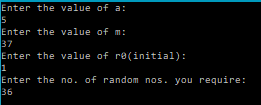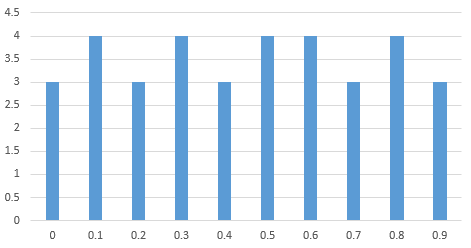Distribution of Random Numbers in windows of 0.1 after scaling down to [0,1]
0.000000 3 0.100000 4 0.200000 3 0.300000 4 0.400000 3 0.500000 4 0.600000 4 0.700000 3 0.800000 4 0.900000 3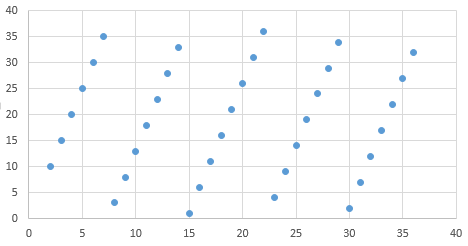Correlation check of r_{i+1} vs r_{i} for a=5 and m=37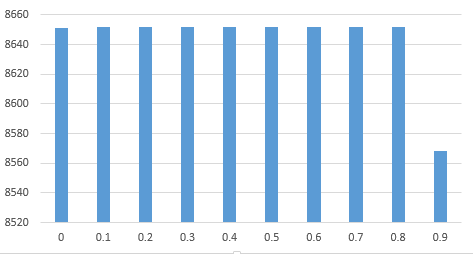Distribution of Random Numbers in windows of 0.1 after scaling down to [0,1]
0.000000 8651 0.100000 8652 0.200000 8652 0.300000 8652 0.400000 8652 0.500000 8652 0.600000 8652 0.700000 8652 0.800000 8652 0.900000 8568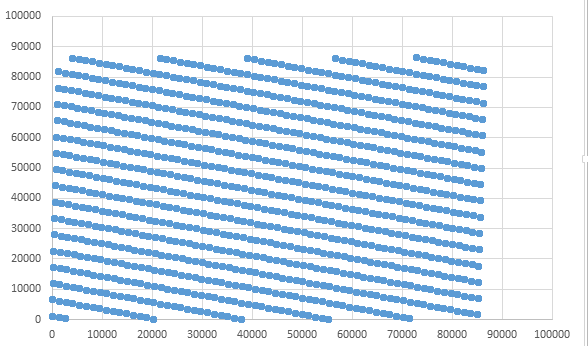Correlation check of r_{i+1} vs r_{i} for a=1093 and m=86436

So, we can see that both the pairs of values of a and m failed the correlation test and the distribution tests weren’t ideal either.

That is why mathematicians, spend a lot of time in choosing the correct set of values. Now, there is one set of value that is known to be pass the above tests, but I couldn’t verify it as the numbers were very large, and my program couldn’t handle these. The values are: a=16807 and m=2147483647 suggested by Par and Miller, who spent over 30 years surveying a large number of random number generators.

But now let me modify the above mentioned algorithm a little bit. Let’s add an offset parameter c.
So that the formula looks like:$r_{i+1}=(a\times r_{i} +c ) \mathrm{mod } m$

Now, let’s modify the above program to use this new formula and perform the above checks.

### CODE:

/********************************************
*********RANDOM NUMBER GENERATOR*************
***GENERATE RANDOM NUMBER USING (ari+c)mod m****
********************************************/
#include<stdio.h>
#include<math.h>
/**Function that generates a random number.
Parameters:
r0: initial (first) seed
a: scale factor , so that a*r0 give the first random number
m: gives the max. value of random numbers that can be generated (m-1)
c: additional displacement factor
**/
int rand(int r0, int a, int m, int c){
int r1=(a*r0+c)%m;
return r1;
}
/**Function that generates random numbers given a seed, and stores them in an array that is passed as an argument.
Parameters:
r0: initial (first) seed
a: scale factor , so that a*r0 give the first random number
m: gives the max. value of random numbers that can be generated (m-1)
c: additional displacement factor
n: no. of random numbers to be generated
x[n]: array that will store the random numbers
**/
void randomNos(int r0, int a, int m, int c, int n, int x[n]){
int r1=rand(r0,a,m,c);;
int i;
for(i=0;i<n;i++){
x[i]=r1;
r1=rand(r1,a,m,c);
}
}
main(){
int a, m, c, r0, n;
printf("Enter the value of a:\n");
scanf("%d",&a);
printf("Enter the value of m:\n");
scanf("%d",&m);
printf("Enter the value of c:\n");
scanf("%d",&c);
printf("Enter the value of r0(initial):\n");
scanf("%d",&r0);
printf("Enter the no. of random nos. you require:\n");
scanf("%d",&n);
int randNos[n];
randomNos(r0, a, m, c, n, randNos);

//Renormalize the randomnumbers so that their values are from within [0,1]
int i;
double randNosNew[n];
for(i=0;i<n;i++){
randNosNew[i]=(double)randNos[i]/(m-1);
}

//Begin distribution calculations within different intervals
int j;
double h=0.1; //width of interval
int count; //10 intervals of width 0.1
for(j=0;j<10;j++){
count[j]=0;
for(i=0;i<n;i++){
//find out the number of randomnumbers within an interval
if((j*h<=randNosNew[i])&&(randNosNew[i]<(j+1)*h)){
count[j]++;  //find out the number of randomnumbers within an interval
}
}
}

FILE *fp="NULL";
fp=fopen("randNosDistribution.txt","w");
for(i=0;i<10;i++){
fprintf(fp,"%lf\t%d\n",i*h,count[i]);
}
//Correlation Checks
//Store r_{i} & r_{i+1} in a file and plot them to check for correlation
FILE *fp1="NULL";
fp1=fopen("randNosCorrelation.txt","w");
for(i=0;i<n-1;i++){
fprintf(fp1,"%d\t%d\n",randNos[i],randNos[i+1]);

}
}


### OUTPUT:

Try the following values of a=1093, m=86436 and c=18257
and plot the distribution and correlation.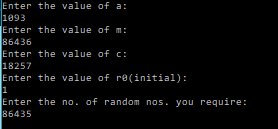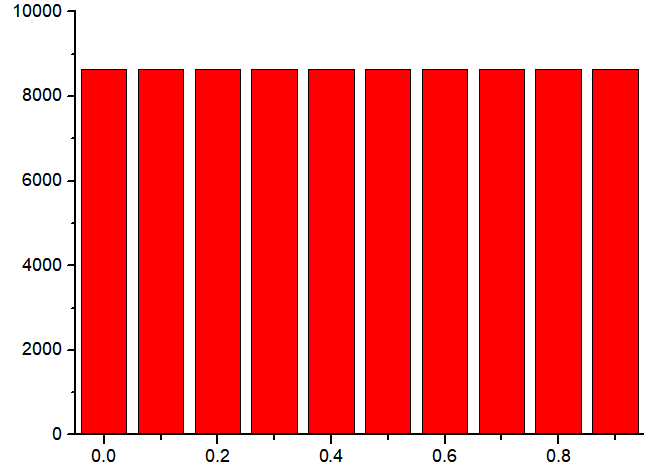Distribution of Random Numbers in windows of 0.1 after scaling down to [0,1]
0.000000 8643 0.100000 8643 0.200000 8644 0.300000 8643 0.400000 8644 0.500000 8644 0.600000 8643 0.700000 8643 0.800000 8644 0.900000 8643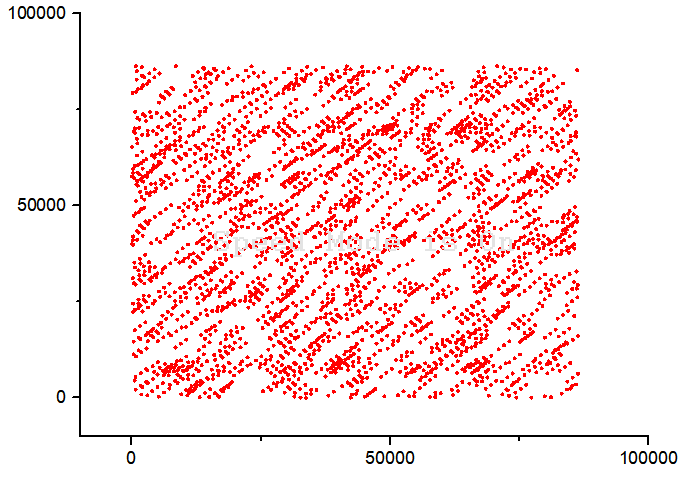Correlation check of r_{i+1} vs r_{i} for a=1093 and m=86436 and c=18257

Finally we see that the above set of values passes our checks, and hence would serve the purpose of use in our programs involving random number generation.

From now on, in future posts on random numbers applications, I will probably be using this new formula and the above set of values.

It must be noted that the above checks and tests are not a sufficient to check our random number generator, as we will se in later posts. Therefore, it is often useful to try to model some real-life random process whose properties and behaviour are already known and well studied, and see if the random number generator is able to correctly reproduce that or not.

### References and Resources:

https://en.wikipedia.org/wiki/Cryptographically_secure_pseudorandom_number_generator
Numerical Recipes in C[wpedon id="7041" align="center"]